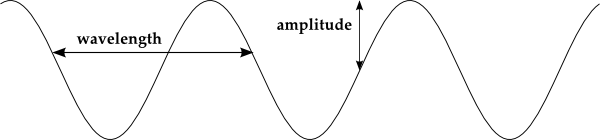HomeNAAP LabsHydrogen Energy Levels › Light

# Light

## Properties of Light

We are all familiar with light. Through light we see the world. But what is it? Light can be thought of as “particles” of electromagnetic energy called photons. Photons exhibit many properties that we are familiar with as particles. But light also exhibits wavelike properties (which property – wave or particle – it will manifest depends on how the light is being observed). It is frequently convenient to express the properties of the light in wave terminology.Components of a Wave

For light, the relationship between wavelength (λ) and frequency (ƒ) is straightforward, their product is equal to the speed of the wave, which for light is (c ≈ 3.00 × 108 m/s). That is

 c = λ · ƒ

Another relationship that was instrumental in the formation of quantum mechanics was the relationship between the energy of a photon at a certain frequency. It is

 E = h · ƒ

where h = 6.626 × 10-34 J·s is called Planck's constant. Since the energy goes up as the frequency increases, the energy is directly proportional to the frequency.

Because frequency and wavelength are related by a constant (c) the energy can also be written in terms of wavelength: E = h · c / λ. When the energy increases the wavelength decreases and vice versa. That is, energy in inversely proportional to wavelength.

In short, a photon can be described by either its energy, frequency, or wavelength. All three methods are frequently used.The full spectrum of light

## Electromagnetic Spectrum

Light can have a large range of values of energy/wavelength/frequency covering what is called the electromagnetic spectrum. We often split the full spectrum into smaller regions and talk about the different “kinds” of light. Radio waves are the least energetic kind of photons while gamma rays are the most energetic kind of photons. The figure below shows the electromagnetic spectrum. Note how small of a region the visible light we see with the human eye covers.